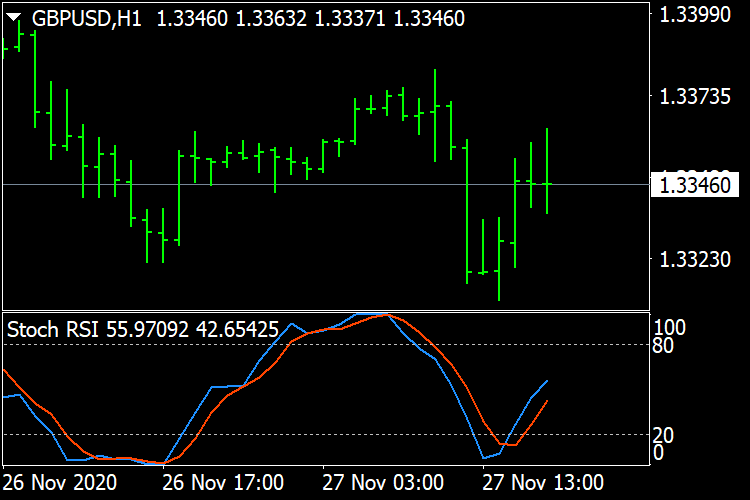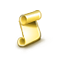Interesting script?
So post a link to it -
let others appraise it
You liked the script? Try it in the MetaTrader 5 terminal# Stoch RSI MT4 - indicator for MetaTrader 4

Views:
6020
Rating:
Published:
2020.11.27 16:58
Updated:
2020.11.27 19:06

The Stochastic RSI indicator (Stoch RSI) is essentially an indicator of an indicator. It is used in technical analysis to provide a stochastic calculation to the RSI indicator. This means that it is a measure of RSI relative to its own high/low range over a user defined period of time. The Stochastic RSI is an oscillator that calculates a value between 0 and 1 which is then plotted as a line. This indicator is primarily used for identifying overbought and oversold conditions.Fibonacci Potential Entry - MT4

The 8 effective steps to build a robust day trading plan using Fibonacci retracementDoulble MA Cross Draw Histogram

Use the intersection of two moving average lines to draw a vertical lineIdentify potential Trends/Range (Pivot Points) - MT4

This script was developed in order to help us detect if a chart is actually trending or going nowhere.AverageRangeLines_v1.6